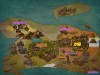2017-08-24 16:20:37

## 此题不需要代码很长的模拟。

### ⑦ 代码

#include<cstdio>
#include<iostream>
#include<cmath>
#include<cstring>
#define f(i,a,b) for(register int i=a;i<=b;++i)
bool flag,havenum;
char s,ansx;
int son,mother,pre,lor=1,equal;
int main()
{
int len;
scanf("%s",s+1);
s[pre]='+';len=strlen(s+1);s[len+1]='+';//随便一个符号都可以
f(i,1,len+1)
{
if(s[i]>='a'&&s[i]<='z')ansx=s[i];
if(s[i]=='='||s[i]=='-'||s[i]=='+')
{
havenum=0;
if(i==1||i-1==equal){pre=i;continue;}
int j,x=0;
if(isdigit(s[i-1]))flag=0;
else flag=1;
for(j=pre+1;j<=i-1-flag;++j)
{x=(x<<1)+(x<<3)+(s[j]^'0');havenum=1;}
if(havenum==0)x=1;
if(flag==0)son+=x*lor*-1*(s[pre]-44)*-1;
else mother+=x*lor*(s[pre]-44)*-1;//ASCII - 45 + 43
//printf("%d %d %d %d\n",i,x,son,mother);
pre=i;
if(s[i]=='=')lor=-1,s[i]='+',equal=i;
}
}double ansy=double(son)/double(mother);if(fabs(ansy)-0.0<0.000001)ansy=0.0;
printf("%c=%.3lf",ansx,ansy);return 0;
}
• star
首页